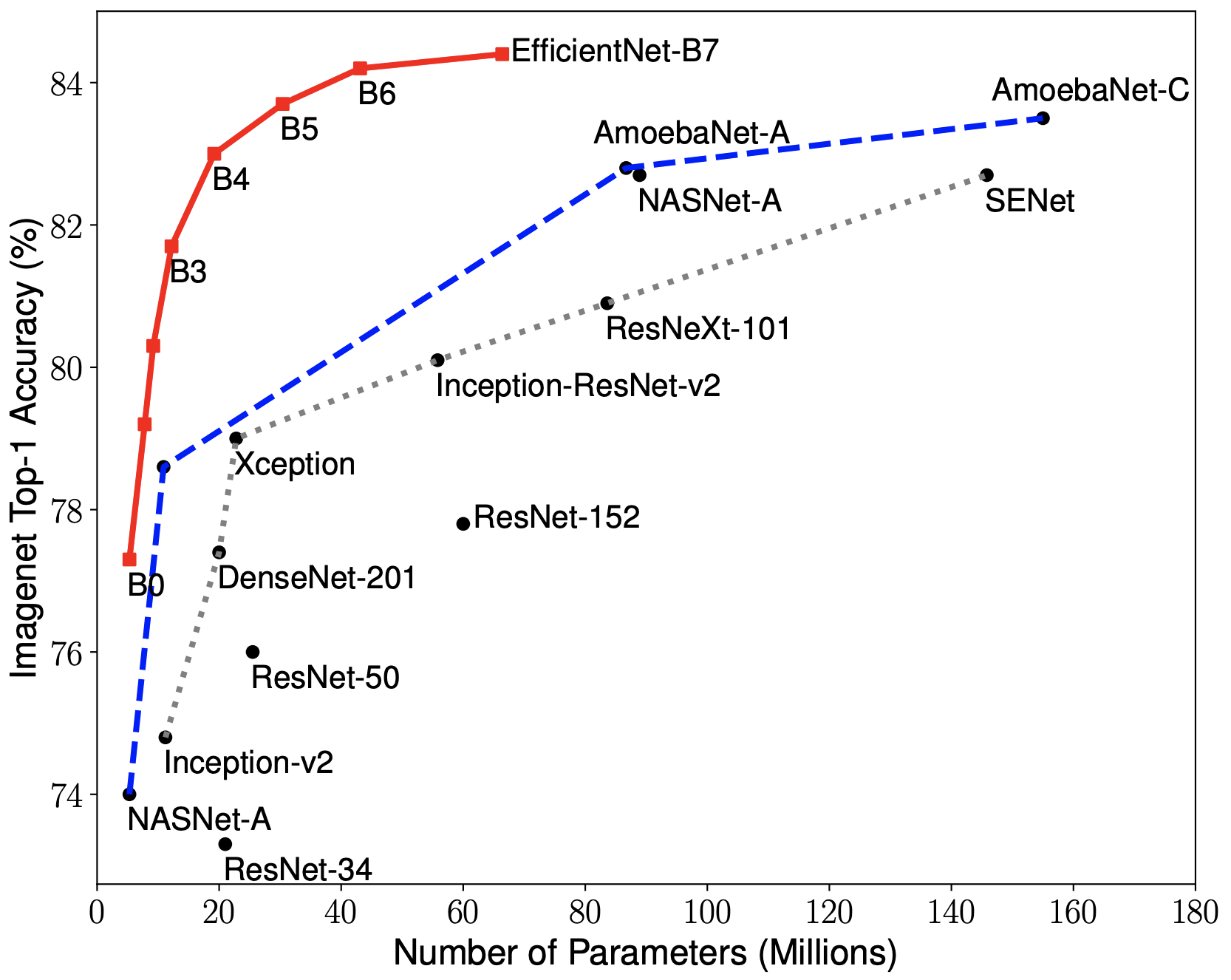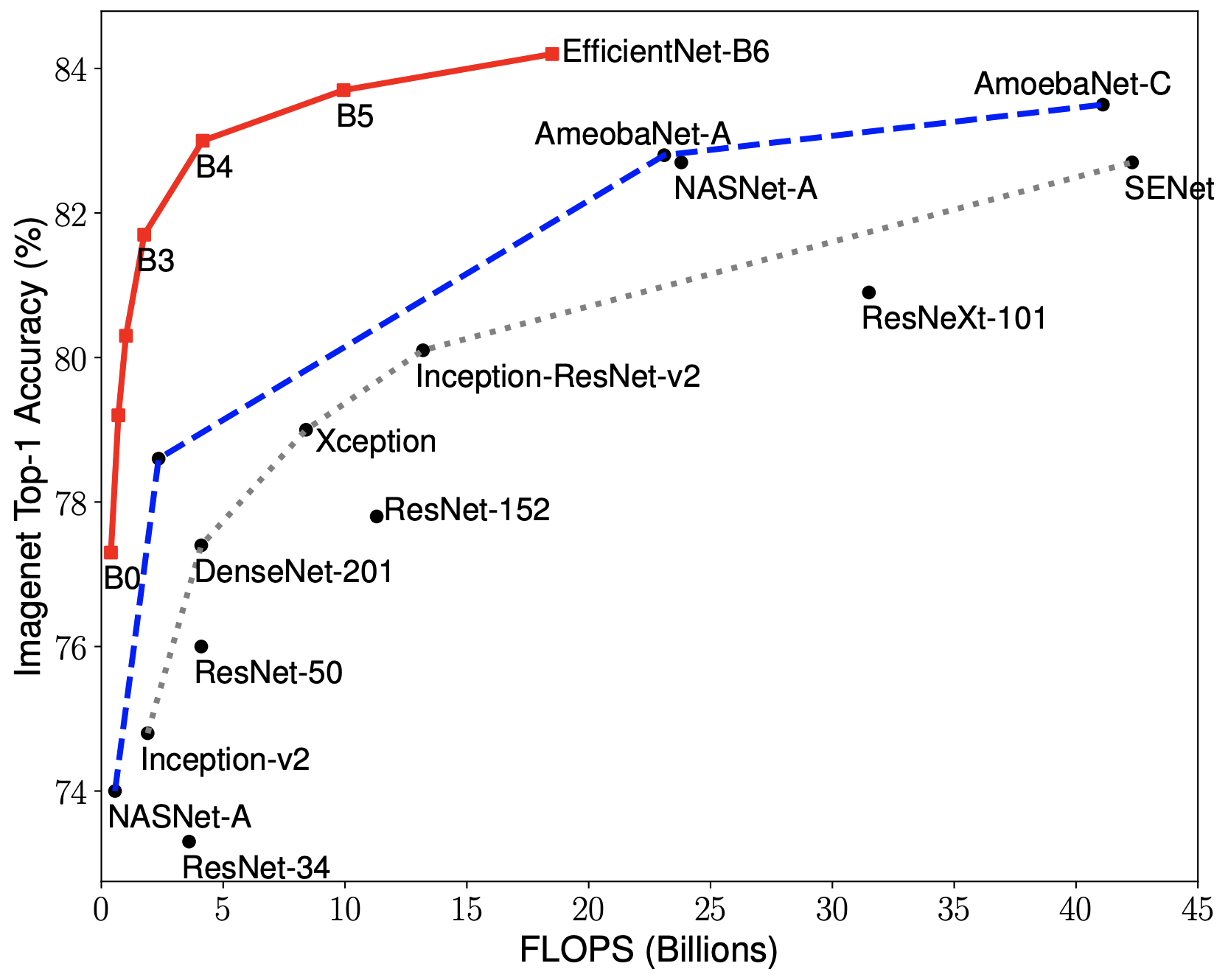## EfficientNets in Keras

Keras implementation of EfficientNets from the paper EfficientNet: Rethinking Model Scaling for Convolutional Neural Networks.

Contains code to build the EfficientNets B0-B7 from the paper, and includes weights for configurations B0-B3. B4-B7 weights will be ported when made available from the Tensorflow repository.

Supports building any other configuration model of efficient nets as well, other than the B0-B7 variants.

# Efficient Nets and Compound Coefficeint Scaling

The core idea about Efficient Nets is the use of compound scaling - using a weighted scale of three inter-connected hyper parameters of the model - Resolution of the input, Depth of the Network and Width of the Network.

When phi, the compound coefficient, is initially set to 1, we get the base configuration - in this case EfficientNetB0. We then use this configuration in a grid search to find the coefficients alpha, beta and gamma which optimize the following objective under the constraint:

Once these coefficients for alpha, beta and gamma are found, then simply scale phi, the compound coeffieints by different amounts to get a family of models with more capacity and possibly better performance.

In doing so, and using Neural Architecture Search to get the base configuration as well as great coefficients for the above, the paper generates EfficientNets, which outperform much larger and much deeper models while using less resources during both training and evaluation.# Installation

## From PyPI:

\$ pip install keras_efficientnets

## From Master branch:

pip install git+https://github.com/titu1994/keras-efficientnets.git

OR

git clone https://github.com/titu1994/keras-efficientnets.git
cd keras-efficientnets
pip install .


# Usage

Simply import keras_efficientnets and call either the model builder EfficientNet or the pre-built versions EfficientNetBX where X ranger from 0 to 7.

from keras_efficientnets import EfficientNetB0

model = EfficientNetB0(input_size, classes=1000, include_top=True, weights='imagenet')


To construct custom EfficientNets, use the EfficientNet builder. The EfficientNet builder code requires a list of BlockArgs
as input to define the structure of each block in model. A default set of BlockArgs are provided in keras_efficientnets.config.

from keras_efficientnets import EfficientNet, BlockArgs

block_args_list = [
# First number is input_channels, second is output_channels.
BlockArgs(32, 16, kernel_size=3, strides=(1, 1), num_repeat=1, se_ratio=0.25, expand_ratio=1),
BlockArgs(16, 24, kernel_size=3, strides=(2, 2), num_repeat=2, se_ratio=0.25, expand_ratio=6),
...
]

model = EfficientNet(input_shape, block_args_list, ...)


# Requirements

• Tensorflow 1.13+ (CPU or GPU version must be installed before installation of this library)
• Keras 2.2.4+
 Mingxing Tan and Quoc V. Le. EfficientNet: Rethinking Model Scaling for Convolutional Neural Networks. ICML 2019. Arxiv link: https://arxiv.org/abs/1905.11946.